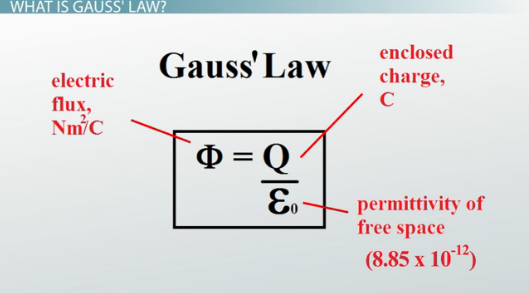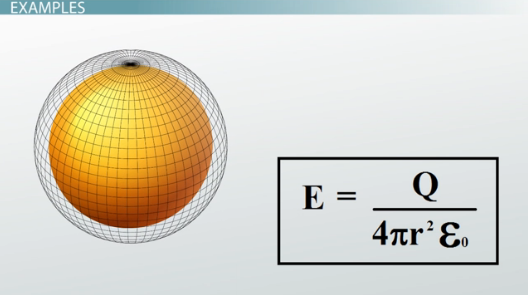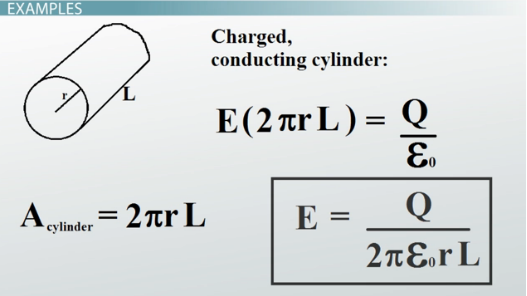# Gauss' Law: Definition & Examples

An error occurred trying to load this video.

Try refreshing the page, or contact customer support.

Coming up next: Electric Field & the Movement of Charge

### You're on a roll. Keep up the good work!

Replay
Your next lesson will play in 10 seconds
• 0:05 What is Gauss' Law?
• 1:40 Examples of Gauss' Law
• 3:20 Practice Problem
• 4:20 Lesson Summary

Want to watch this again later?

Timeline
Autoplay
Autoplay
Speed

#### Recommended Lessons and Courses for You

Lesson Transcript
Instructor: David Wood

David has taught Honors Physics, AP Physics, IB Physics and general science courses. He has a Masters in Education, and a Bachelors in Physics.

After watching this video, you will be able to explain what Gauss' Law is, derive Gauss' Law for a sphere and cylinder, and use it to solve problems. A short quiz will follow.

## What Is Gauss' Law?

Gauss' Law is a law that describes what an electric field will look like due to a known distribution of electric charge. It was first formulated in the 19th century. Gauss' Law also comprises one of the four Maxwell's Equations that describe the force of electromagnetism.

To be more specific, Gauss' Law can be explained in words as: the total of the electric flux out of a closed surface is equal to the magnitude of the charge enclosed divided by the permittivity of free space. As a mathematical equation, it looks like this:Q is the charge enclosed by a surface, epsilon-zero is the permittivity of free space, which is just a constant that is always equal to 8.85 x 10^-12, and phi is the electric flux through the surface.

But what is electric flux? The electric flux, represented by the Greek letter phi, is the electric field, E, multiplied by the area, A, of a surface perpendicular to the field. Phi equals EA. It's basically the number of field lines that pass through a surface -- more field lines means a larger flux.

So for example, you could use Gauss' Law to figure out the electric field created by a charged conducting sphere. In that case, you have a charge surrounded by a spherical surface. If you wanted to know the total flux, you would take the electric field strength at the surface of the sphere, and multiply it by the surface area of the sphere.

But not all surfaces are spheres. So the exact equation for Gauss' Law varies depending on the particular surface you're looking at.

## Examples

This might be easier if we went through a few examples. Let's first go back to the sphere.

Gauss' law tells us that the flux is equal to the charge Q, over the permittivity of free space, epsilon-zero. But flux is also equal to the electric field E multiplied by the area of the surface A. So EA equals Q over epsilon-zero.

For a sphere, the surface area is given by 4pir^2, so we can plug that in for A. And finally, if we rearrange for the electric field E, we find that the electric field Es is equal to Q over 4piepsilon-zeror^2. This is an expression for the electric field created by a charged sphere.Okay, let's try another one. How about a conducting cylinder?

We start with the same basic equation. But this time, the area is different. The surface area of a cylinder is equal to 2pirL, where r is the radius of the cross-section of the cylinder and L is the length of the cylinder. If we rearrange that for E again, this time we get Q over 2piepsilon-zerorL. So that's an expression for the electric field created by a charged cylinder.We could do the same thing for any uniformly shaped object, so Gauss' Law is a very powerful tool.

To unlock this lesson you must be a Study.com Member.

### Register to view this lesson

Are you a student or a teacher?

### Unlock Your Education

#### See for yourself why 30 million people use Study.com

##### Become a Study.com member and start learning now.
Back
What teachers are saying about Study.com

### Earning College Credit

Did you know… We have over 160 college courses that prepare you to earn credit by exam that is accepted by over 1,500 colleges and universities. You can test out of the first two years of college and save thousands off your degree. Anyone can earn credit-by-exam regardless of age or education level.